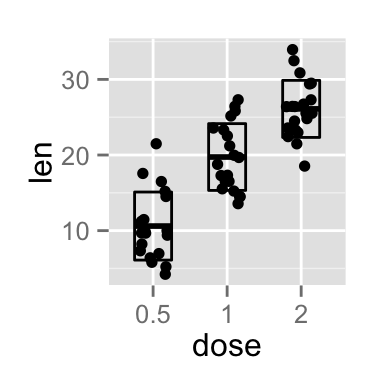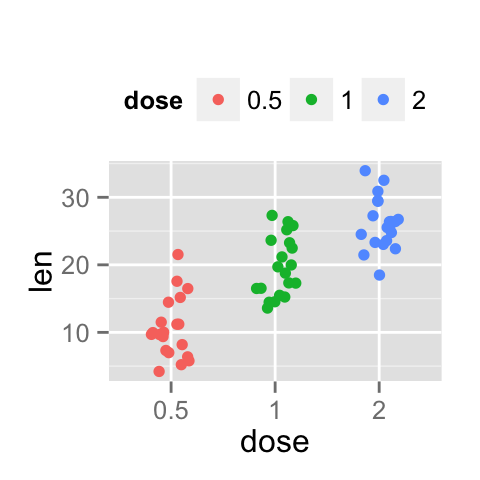# ggplot2 stripchart (jitter) : Quick start guide - R software and data visualization

This R tutorial describes how to create a stripchart using R software and ggplot2 package. Stripcharts are also known as one dimensional scatter plots. These plots are suitable compared to box plots when sample sizes are small.

The function geom_jitter() is used.# Prepare the data

ToothGrowth data sets are used :

``````# Convert the variable dose from a numeric to a factor variable
ToothGrowth\$dose <- as.factor(ToothGrowth\$dose)
``````##    len supp dose
## 1  4.2   VC  0.5
## 2 11.5   VC  0.5
## 3  7.3   VC  0.5
## 4  5.8   VC  0.5
## 5  6.4   VC  0.5
## 6 10.0   VC  0.5``````

Make sure that the variable dose is converted as a factor variable using the above R script.

# Basic stripcharts

``````library(ggplot2)
# Basic stripchart
ggplot(ToothGrowth, aes(x=dose, y=len)) +
geom_jitter()
# Change the position
# 0.2 : degree of jitter in x direction
p<-ggplot(ToothGrowth, aes(x=dose, y=len)) +
geom_jitter(position=position_jitter(0.2))
p
# Rotate the stripchart
p + coord_flip()``````Choose which items to display :

``p + scale_x_discrete(limits=c("0.5", "2"))``Change point shapes and size :

``````# Change point size
ggplot(ToothGrowth, aes(x=dose, y=len)) +
geom_jitter(position=position_jitter(0.2), cex=1.2)
# Change shape
ggplot(ToothGrowth, aes(x=dose, y=len)) +
geom_jitter(position=position_jitter(0.2), shape=17)``````Read more on point shapes : ggplot2 point shapes

# Add summary statistics on a stripchart

The function stat_summary() can be used to add mean/median points and more to a stripchart.

## Add mean and median points

``````# stripchart with mean points
p + stat_summary(fun.y=mean, geom="point", shape=18,
size=3, color="red")
# stripchart with median points
p + stat_summary(fun.y=median, geom="point", shape=18,
size=3, color="red")``````## Stripchart with box blot and violin plot

``````# Add basic box plot
ggplot(ToothGrowth, aes(x=dose, y=len)) +
geom_boxplot()+
geom_jitter(position=position_jitter(0.2))
ggplot(ToothGrowth, aes(x=dose, y=len)) +
geom_boxplot(notch = TRUE)+
geom_jitter(position=position_jitter(0.2))
ggplot(ToothGrowth, aes(x=dose, y=len)) +
geom_violin(trim = FALSE)+
geom_jitter(position=position_jitter(0.2))``````Read more on box plot : ggplot2 box plot

Read more on violin plot : ggplot2 violin plot

## Add mean and standard deviation

The function mean_sdl is used. mean_sdl computes the mean plus or minus a constant times the standard deviation.

In the R code below, the constant is specified using the argument mult (mult = 1). By default mult = 2.

The mean +/- SD can be added as a crossbar or a pointrange :

``````p <- ggplot(ToothGrowth, aes(x=dose, y=len)) +
geom_jitter(position=position_jitter(0.2))
p + stat_summary(fun.data="mean_sdl", mult=1,
geom="crossbar", width=0.5)
p + stat_summary(fun.data=mean_sdl, mult=1,
geom="pointrange", color="red")``````Note that, you can also define a custom function to produce summary statistics as follow

``````# Function to produce summary statistics (mean and +/- sd)
data_summary <- function(x) {
m <- mean(x)
ymin <- m-sd(x)
ymax <- m+sd(x)
return(c(y=m,ymin=ymin,ymax=ymax))
}``````

Use a custom summary function :

``p + stat_summary(fun.data=data_summary, color="blue")``# Change point shapes by groups

In the R code below, point shapes are controlled automatically by the variable dose.

You can also set point shapes manually using the function scale_shape_manual()

``````# Change point shapes by groups
p<-ggplot(ToothGrowth, aes(x=dose, y=len, shape=dose)) +
geom_jitter(position=position_jitter(0.2))
p
# Change point shapes manually
p + scale_shape_manual(values=c(1,17,19))``````Read more on point shapes : ggplot2 point shapes

# Change stripchart colors by groups

In the R code below, point colors of the stripchart are automatically controlled by the levels of dose :

``````# Use single color
ggplot(ToothGrowth, aes(x=dose, y=len)) +
geom_jitter(position=position_jitter(0.2), color="red")
# Change stripchart colors by groups
p<-ggplot(ToothGrowth, aes(x=dose, y=len, color=dose)) +
geom_jitter(position=position_jitter(0.2))
p``````It is also possible to change manually stripchart colors using the functions :

• scale_color_manual() : to use custom colors
• scale_color_brewer() : to use color palettes from RColorBrewer package
• scale_color_grey() : to use grey color palettes
``````# Use custom color palettes
p+scale_color_manual(values=c("#999999", "#E69F00", "#56B4E9"))
# Use brewer color palettes
p+scale_color_brewer(palette="Dark2")
# Use grey scale
p + scale_color_grey() + theme_classic()``````Read more on ggplot2 colors here : ggplot2 colors

# Change the legend position

``````p + theme(legend.position="top")
p + theme(legend.position="bottom")
p + theme(legend.position="none")# Remove legend``````The allowed values for the arguments legend.position are : “left”,“top”, “right”, “bottom”.

Read more on ggplot legends : ggplot2 legend

# Change the order of items in the legend

The function scale_x_discrete can be used to change the order of items to “2”, “0.5”, “1” :

``p + scale_x_discrete(limits=c("2", "0.5", "1"))``# Stripchart with multiple groups

``````# Change stripchart colors by groups
ggplot(ToothGrowth, aes(x=dose, y=len, color=supp)) +
geom_jitter(position=position_jitter(0.2))
# Change the position : interval between stripchart of the same group
p<-ggplot(ToothGrowth, aes(x=dose, y=len, color=supp, shape=supp)) +
geom_jitter(position=position_dodge(0.8))
p``````Change stripchart colors and add box plots :

``````# Change colors
p+scale_color_manual(values=c("#999999", "#E69F00", "#56B4E9"))
ggplot(ToothGrowth, aes(x=dose, y=len, color=supp)) +
geom_boxplot(color="black")+
geom_jitter(position=position_jitter(0.2))
# Change the position
ggplot(ToothGrowth, aes(x=dose, y=len, color=supp)) +
geom_boxplot(position=position_dodge(0.8))+
geom_jitter(position=position_dodge(0.8))``````# Customized stripcharts

``````# Basic stripchart
ggplot(ToothGrowth, aes(x=dose, y=len)) +
geom_boxplot()+
geom_jitter(position=position_jitter(0.2))+
labs(title="Plot of length  by dose",x="Dose (mg)", y = "Length")+
theme_classic()
# Change color/shape by groups
p <- ggplot(ToothGrowth, aes(x=dose, y=len, color=dose, shape=dose)) +
geom_jitter(position=position_jitter(0.2))+
labs(title="Plot of length  by dose",x="Dose (mg)", y = "Length")
p + theme_classic()``````Change colors manually :

``````# Continuous colors
p + scale_color_brewer(palette="Blues") + theme_classic()
# Discrete colors
p + scale_color_brewer(palette="Dark2") + theme_minimal()
p + scale_color_brewer(palette="RdBu")``````Read more on ggplot2 colors here : ggplot2 colors

# Infos

This analysis has been performed using R software (ver. 3.1.2) and ggplot2 (ver. 1.0.0)